# tidygenomics

Tidy Verbs for Dealing with Genomic Data Frames

## Description

Handle genomic data within data frames just as you would with `GRanges`. This packages provides method to deal with genomics intervals the “tidy-way” which makes it simpler to integrate in the the general data munging process. The API is inspired by the popular bedtools and the genome_join() method from the fuzzyjoin package.

## Installation

``devtools::install_github("Artjom-Metro/tidygenomics")``

## Documentation

#### genome_intersect

Joins 2 data frames based on their genomic overlap. Unlike the `genome_join` function it updates the boundaries to reflect the overlap of the regions.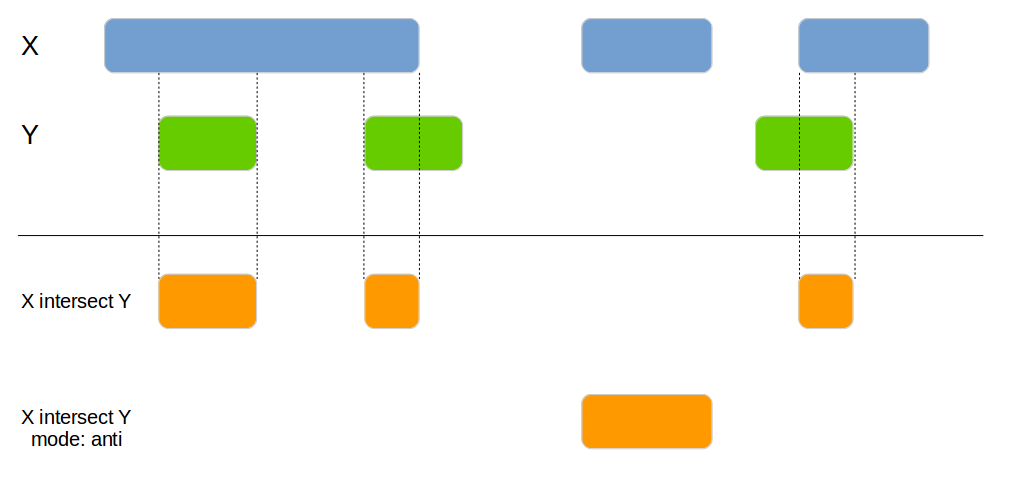``````x1 <- data.frame(id = 1:4,
chromosome = c("chr1", "chr1", "chr2", "chr2"),
start = c(100, 200, 300, 400),
end = c(150, 250, 350, 450))

x2 <- data.frame(id = 1:4,
chromosome = c("chr1", "chr2", "chr2", "chr1"),
start = c(140, 210, 400, 300),
end = c(160, 240, 415, 320))

genome_intersect(x1, x2, by=c("chromosome", "start", "end"), mode="both")``````
id.x chromosome id.y start end
1 chr1 1 140 150
4 chr2 3 400 415

#### genome_subtract

Subtracts one data frame from the other. This can be used to split the x data frame into smaller areas.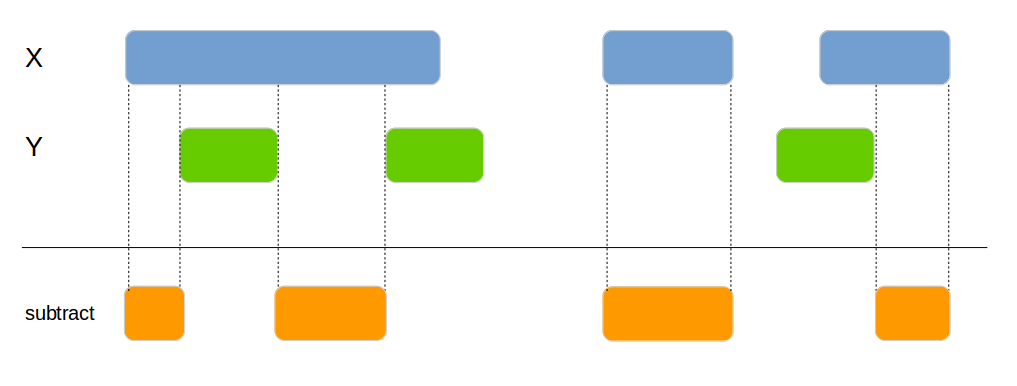``````x1 <- data.frame(id = 1:4,
chromosome = c("chr1", "chr1", "chr2", "chr1"),
start = c(100, 200, 300, 400),
end = c(150, 250, 350, 450))

x2 <- data.frame(id = 1:4,
chromosome = c("chr1", "chr2", "chr1", "chr1"),
start = c(120, 210, 300, 400),
end = c(125, 240, 320, 415))

genome_subtract(x1, x2, by=c("chromosome", "start", "end"))``````
id chromosome start end
1 chr1 100 119
1 chr1 126 150
2 chr1 200 250
3 chr2 300 350
4 chr1 416 450

#### genome_join_closest

Joins 2 data frames based on their genomic location. If no exact overlap is found the next closest interval is used.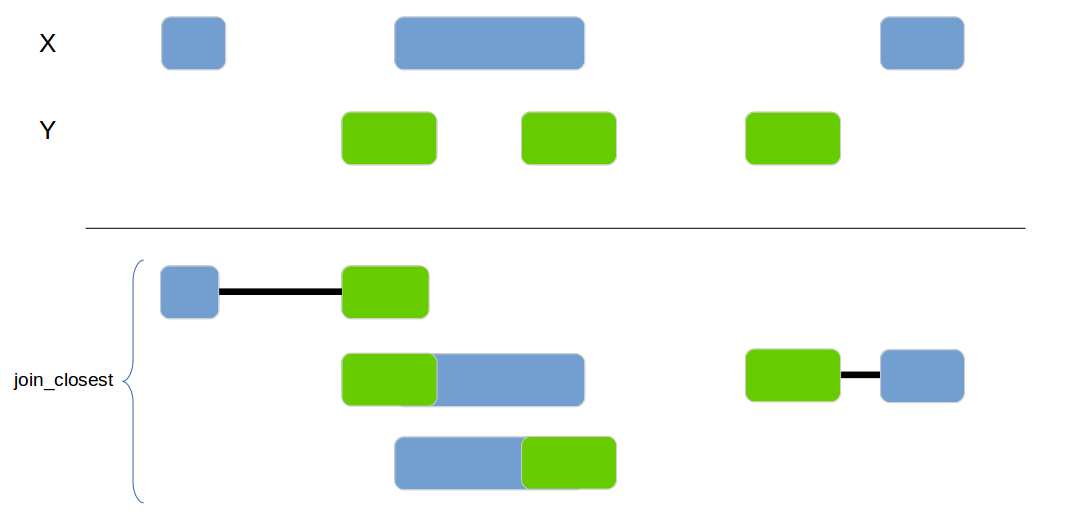``````x1 <- data_frame(id = 1:4,
chr = c("chr1", "chr1", "chr2", "chr3"),
start = c(100, 200, 300, 400),
end = c(150, 250, 350, 450))

x2 <- data_frame(id = 1:4,
chr = c("chr1", "chr1", "chr1", "chr2"),
start = c(220, 210, 300, 400),
end = c(225, 240, 320, 415))
genome_join_closest(x1, x2, by=c("chr", "start", "end"), distance_column_name="distance", mode="left")``````
id.x chr.x start.x end.x id.y chr.y start.y end.y distance
1 chr1 100 150 2 chr1 210 240 59
2 chr1 200 250 1 chr1 220 225 0
2 chr1 200 250 2 chr1 210 240 0
3 chr2 300 350 4 chr2 400 415 49
4 chr3 400 450 NA NA NA NA NA

#### genome_cluster

Add a new column with the cluster if 2 intervals are overlapping or are within the `max_distance`.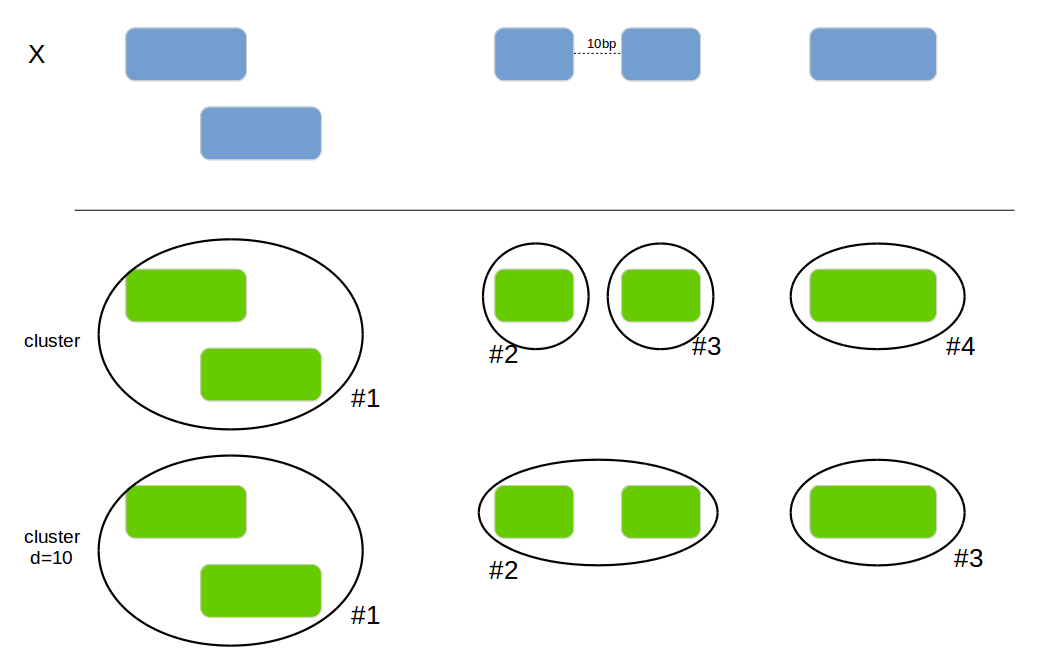``````x1 <- data.frame(id = 1:4, bla=letters[1:4],
chromosome = c("chr1", "chr1", "chr2", "chr1"),
start = c(100, 120, 300, 260),
end = c(150, 250, 350, 450))
genome_cluster(x1, by=c("chromosome", "start", "end"))``````
id bla chromosome start end cluster_id
1 a chr1 100 150 0
2 b chr1 120 250 0
3 c chr2 300 350 2
4 d chr1 260 450 1
``genome_cluster(x1, by=c("chromosome", "start", "end"), max_distance=10)``
id bla chromosome start end cluster_id
1 a chr1 100 150 0
2 b chr1 120 250 0
3 c chr2 300 350 1
4 d chr1 260 450 0

#### genome_complement

Calculates the complement of a genomic region.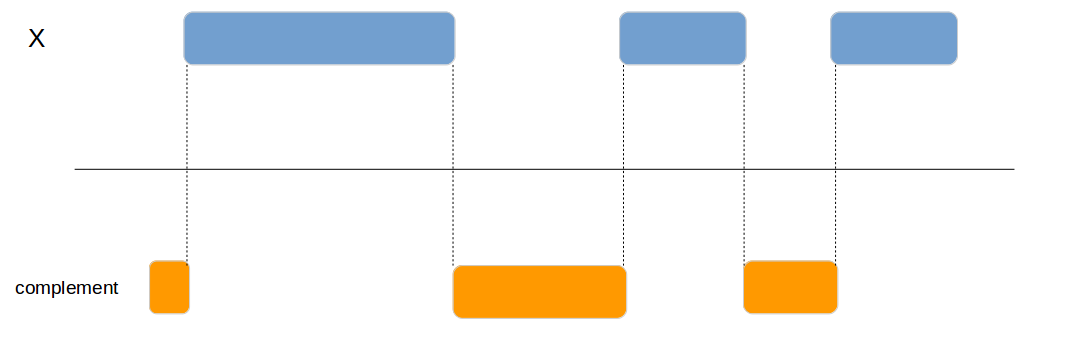``````x1 <- data.frame(id = 1:4,
chromosome = c("chr1", "chr1", "chr2", "chr1"),
start = c(100, 200, 300, 400),
end = c(150, 250, 350, 450))

genome_complement(x1, by=c("chromosome", "start", "end"))``````
chromosome start end
chr1 1 99
chr1 151 199
chr1 251 399
chr2 1 299

#### genome_join

Classical join function based on the overlap of the interval. Implemented and mainted in the fuzzyjoin package and documented here only for completeness.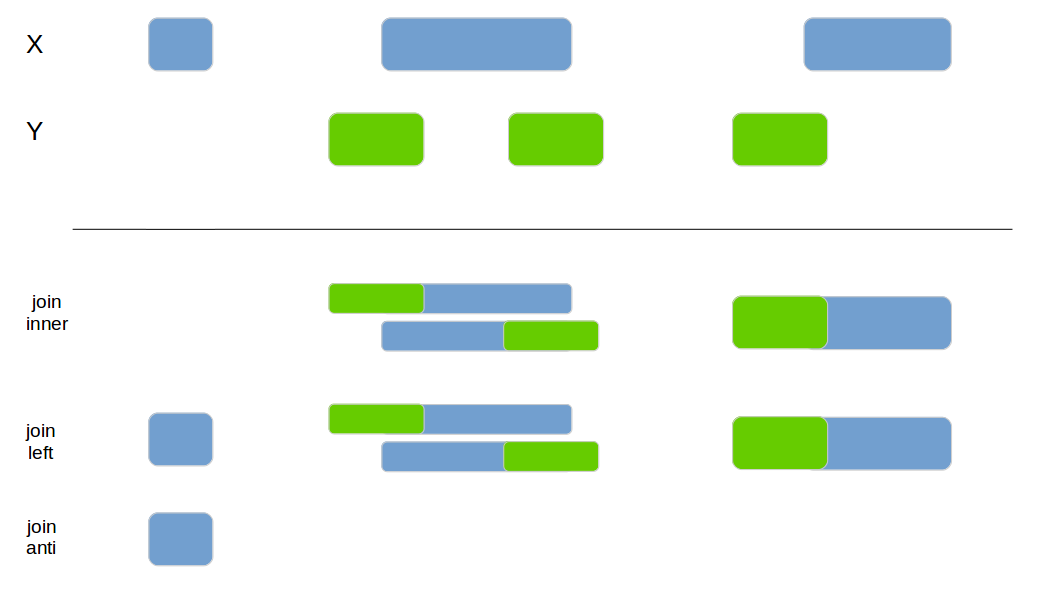``````x1 <- data_frame(id = 1:4,
chr = c("chr1", "chr1", "chr2", "chr3"),
start = c(100, 200, 300, 400),
end = c(150, 250, 350, 450))

x2 <- data_frame(id = 1:4,
chr = c("chr1", "chr1", "chr1", "chr2"),
start = c(220, 210, 300, 400),
end = c(225, 240, 320, 415))
fuzzyjoin::genome_join(x1, x2, by=c("chr", "start", "end"), mode="inner")``````
id.x chr.x start.x end.x id.y chr.y start.y end.y
2 chr1 200 250 1 chr1 220 225
2 chr1 200 250 2 chr1 210 240
``fuzzyjoin::genome_join(x1, x2, by=c("chr", "start", "end"), mode="left")``
id.x chr.x start.x end.x id.y chr.y start.y end.y
1 chr1 100 150 NA NA NA NA
2 chr1 200 250 1 chr1 220 225
2 chr1 200 250 2 chr1 210 240
3 chr2 300 350 NA NA NA NA
4 chr3 400 450 NA NA NA NA
``fuzzyjoin::genome_join(x1, x2, by=c("chr", "start", "end"), mode="anti")``
id chr start end
1 chr1 100 150
3 chr2 300 350
4 chr3 400 450

## Inspiration

If you have any additional questions or encounter issues please raise them on the github page.